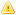Home |Sign in | EnglishHelpWLanguageWLanguage functionsStandard functionsNumeric values management functionsFinancial functionsFinancial functionsRelated examplesSee alsoFinCurrentValFinDecreasingRedemptionFinErrorFinFutureValFinInterestRateFinLinearRedemptionFinNetCurrentValFinPaymentNbFinPeriodInterestFinRedemptionFinRepayValFinancial constantsFinancial functionsThis content has been translated automatically. Click here to view the French version.
WINDEVWEBDEVWINDEV MobileOthersFinancial functionsVersions 17 and laterThese functions are now available for iPhone/iPad applications.New in version 17These functions are now available for iPhone/iPad applications.These functions are now available for iPhone/iPad applications.Versions 21 and laterThese functions are now available in Universal Windows 10 App mode.New in version 21These functions are now available in Universal Windows 10 App mode.These functions are now available in Universal Windows 10 App mode.Versions 23 and laterThese functions are now available for Java applications.These functions are now available for Android applications.These functions are now available in Android Widget mode.New in version 23These functions are now available for Java applications.These functions are now available for Android applications.These functions are now available in Android Widget mode.These functions are now available for Java applications.These functions are now available for Android applications.These functions are now available in Android Widget mode.
The financial functions are as follows:
 FinCurrentVal Returns the current value of investment. FinDecreasingRedemption Calculates the amortization value of a good over a specific period of time, via the formula for fixed-rate decreasing amortization. FinError Returns the number of the last error caused by a Finxxx function (financial functions). FinFutureVal Returns the future value of an investment. FinInterestRate Calculates the interest rate for a loan over a specific period of time with fixed payments. FinLinearRedemption Calculates the value of linear amortization of a good over a specific period of time. FinNetCurrentVal Returns the net current value of investment. FinPaymentNb Returns the number of payments required to pay off a capital according to a given rate. FinPeriodInterest Calculates for a given period the amount of interests due for a loan paid off by fixed periodic payments with a fixed interest rate. FinRedemption Creates an amortization matrix for a fixed-rate loan over a defined period of time. FinRepayVal Returns the amount of each regular payment for an investment with fixed interest rate and fixed payment.

Related Examples:Unit examples (WINDEV): The financial functions [ + ] Using the main functions for performing financial calculations in WINDEV:- Calculate the number of repayments- Calculate the future value of an investmentUnit examples (WINDEV): The financial functions [ + ] Using the main functions for performing financial calculations in WINDEV:- Calculate the number of repayments- Calculate the future value of an investmentComplete examples (WINDEV): WD Loan [ + ] The power of WINDEV and the ease-of-use of WLanguage have allowed us to develop this application within a single day!This example is used to simulate loans and to:- calculate the amount of the monthly repayments from the amount borrowed- calculate the amount that can be borrowed from a given monthly repayment- calculate the income of an investment from a monthly paymentFor each case, you have the ability to view the amortization table corresponding to your parameters.Unit examples (WINDEV Mobile): The Financial functions [ + ] Use of financial functions.This example calculates the number of payments needed to pay back a loan, the total cost of a loan as well as the interests returned by an investment.Complete examples (WEBDEV): WW_Loan [ + ] The power of WEBDEV and the simplicity of the WLanguage allowed us to develop this site in just one day!This example is used to simulate loans and to:- calculate the amount of monthly payments from the amount that was borrowed- calculate the amount that can be borrowed from a given monthly payment- calculate the income of an investment from a monthly depositThe amortization table can be displayed for each one of the cases.This table can be edited in a file in PDF format (standard feature of WEBDEV).